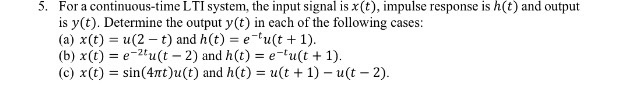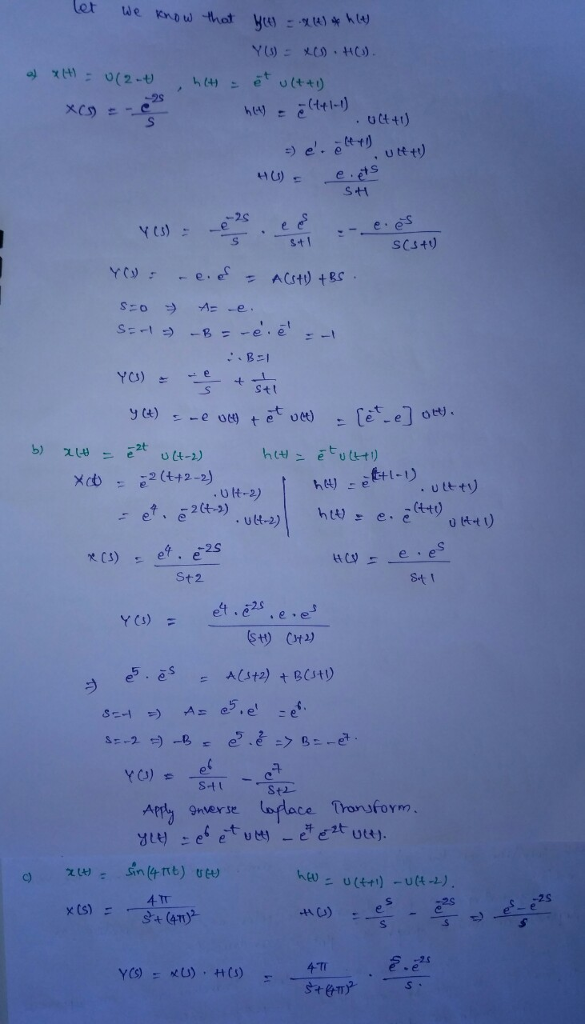# Homework Solution: For a continuous-time LTI system, the input signal is x(t), impulse response is h(t) and output is y(t). Determine the o…For a continuous-time LTI system, the input signal is x(t), impulse response is h(t) and output is y(t). Determine the output y(t) in each of the following cases: (a) x(t) = u (2 - t) and h(t) = e^-t u (t + 1). (b) x(t) = e^-2t u(t - 2) and h(t) = e^-t u (t + 1). (c) x(t) = sin(4 pi t)u(t) and h(t) = u(t + 1) - u(t - 2).